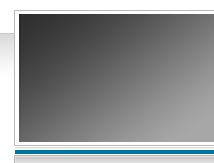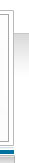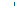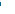ClassicalRealAnalysis.info
Serving the mathematics community.HomeMore Resources

## Selected Real Analysis Resources.CONTENTS

1.  D.R.I.P. Resources.
2.  Calculus  Resource
3.  Elementary Real Analysis  Resources.
5.  etc.
6.  etc.

 1.  D.R.I.P. Resources.   Integration theory by the end of the nineteeth century was in an intellectually messy state.  By the middle of the next century, however, it had matured into a complete and compelling area of mathematics.   The same cannot be said for the state of undergraduate instruction in integration theory.    Even today the standard curriculum would leave the unprepared student  floudering around with concepts and misconceptions that were cleared up long ago.   A typical sequence of courses for the student probably covers the Riemann integral, the improper Riemann integral and little else.  Graduate instruction then abandons that theory and moves directly to the Lebesgue integral.   The DRIP Project D.R.I.P. = Dump-The-Riemann-Integral proposes dropping the Riemann integral from the curriculum.  The replacement suggestions are The Calculus Integral (i.e., Newton's original integral that focusses on differentiable functions) The regulated integral (i.e., basing the theory on step functions and uniform limits of step functions). Dixmier's teaching integral (i.e., focussing on continuous functions differentiable except at finitely many points). Zakon's teaching integral (i.e., focussing on continuous functions differentiable except at countably many points). The Lebesgue integral [but presented in some simpler way for undergraduates]. The Henstock-Kurzweil integral integral.     If you are interested in pursuing these ideas please go to our drip resources page for further advice .

# 2.  Calculus  Resources.

Most calculus books (and the market is flooded with them) do an adequate job of teaching the elements of the calculus.  The courses themselves are addressed mainly to acquiring computational skills related to differentiation and integration, not theoretical skills.

If you are suffering in your calculus studies it is most likely because your algebraic and computational skllls are not up to the task.   Most teachers suggest a return to basics:  what is a function, what is a graph, how does composition of functions work, what are the usual manipulations in algebra, how do exponentials and logarithms work, what are the trigonometric functions?  If you are on this web site you are not looking for help in the calculus, but help at the very next stage.

Your task as a serious student of mathematics is to prepare yourself for the next level: real analysis.  The emphasis shifts from computations to theory and concepts.
1. What are the real numbers?
2. What is a derivative?
3. What is an integral?
4. What does continuity mean?
5. How do you prove statements about these concepts?
6. What deeper properties do integrals and derivatives have?
7. Are continuous functions integrable?
8. Are continuous functions differentiable?
9. What properties does a series of functions have?
10. How do power series behave and what are their properties? Etc.
Your first course in elementary real analysis will pull you through this kind of material.  In advance of taking that course maybe you might wish to read some well-written accounts that will get you started thinking in this new way.  One the best FREE versions available is a classic by a master:

The link will take you to the Wiki page where you can find out to download various editions of this.  This is like going back in a time machine to study with one of the all time masters of the subject.  A good experience before starting into a contemporary textbook!

 3.  Elementary Real Analysis  Resources.   [Under construction ...]   For the moment the only material we are suggesting is our own textbook TBB (Elementary Real Analysis) available for free download on this site.     If you are interested in pursuing these ideas please go to our elementary real analysis resources page   for further advice.

 Advanced Real Analysis Resources. Topic:  The problem of characterizing derivatives.  [Under construction ...] The problem naively is to find some necessary and sufficient condition in order that a function  f: [a,b] --> R  would be the derivative everywhere in [a,b] of some other function.   (For example continuity would be sufficient but certainly not necessary,  Baire class 1 would be necessary but not sufficient.)   The first articulation of this problem was given by W. H. Young in 1911.  Here is his statement of the problem and of what was then known about it:   Recent research [of Lebesgue and Vitali] has provided us with a set of necessary and sufficient conditions that a function may be the indefinite integral.., of an- other function and the way has thus been opened to important developments. The corresponding, much more difficult, problem of determining necessary and sufficient conditions that a function may be a differential coefficient, has barely been mooted; indeed, though we know a number of necessary conditions no set even of sufficient conditions has to my knowledge ever been formulated, except that involved in the obvious statement that a continuous function is a differential coefficient. The necessary conditions in question are of consider- able importance and interest. A function which is a differential coefficient has, in fact, various striking properties. It must be pointwise discontinuous with respect to every perfect set; it can have no discontinuities of the first kind; it assumes in every interval all values between its upper and lower bounds in that interval; its value at every point is one of the limits on both sides of the values in the neighbourhood; its upper and lower bounds, when finite, are unaltered, if we omit the values at any countable set of points; the points at which it is infinite form an inner limiting set of content zero. From these necessary conditions we are able to deduce much valuable information as to when a function is certainly not a differential coefficient . . . . These conditions do not, however, render us any material assistance, even in answering the simple question as to whether the product of two differential coefficients is a differential coefficient, and this not even in the special case in which one of the differential coefficients is a continuous function. To research the problem you should consult at least the following: Andrew M. Bruckner, Differentiation of real functions. Lecture Notes in Math., vol. 659, Springer-Verlag, Berlin and New York, 1978, x + 246 pp. or the more recent edition: Differentiation of real functions. Second edition. CRM Monograph Series 5.  American Mathematical Society, Providence, RI, 1994. xii+195 pp. ISBN: 0-8218-6990-6  Chapter seven contains an entertaining and accessible account of the problem, including the original formulation of the problem by W. H. Young. Andy has updated his discussion of this problem in a 1995 survey article written for the Real Analysis Exchange:  Bruckner, Andrew M.  The problem of characterizing derivatives revisited.  Real Anal. Exchange  21  (1995/96),  no. 1, 112--133.  Since then there have been a few varied expressions of conditions that characterize derivatives in perhaps unusual ways:   On Characterizing Derivatives,  D. Preiss and M. Tartaglia Proceedings of the American Mathematical Society, Vol. 123, No. 8 (Aug., 1995), pp. 2417-2420 Freiling, Chris,   On the problem of characterizing derivatives. Real Anal. Exchange 23 (1997/98), no. 2, 805–812. Brian S. Thomson, On Riemann Sums, Real Analysis Exchange Vol. 37(1), 2011/2012, pp. 1–22 If you have material that you wish to add to this topic discussion please contact us.

## ...

...